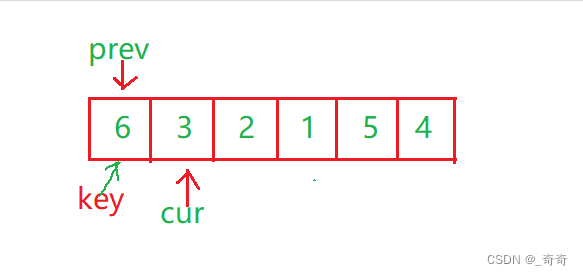﻿ C语言植物大战数据结构快速排序图文示例_C 语言_脚本之家

# C语言植物大战数据结构快速排序图文示例

更新时间：2022年05月10日 17:57:17   作者：_奇奇

“田家少闲月，五月人倍忙”“夜来南风起，小麦覆陇黄”

C语言朱武大战数据结构专栏

C语言植物大战数据结构希尔排序算法

C语言植物大战数据结构堆排序图文示例

C语言植物大战数据结构二叉树递归

## 一、经典1962年Hoare法

```void QuickSort(int* a, int left, int right)
{
//请你完善以下代码
}
int main()
{
int arr[] = {6,1,2,7,9,3,4,5,10,8};
int sz = sizeof(arr) / sizeof(arr);
QuickSort(arr, 0, sz-1);
return 0;
}
```

Hoare法和二叉树的**前序遍历(根 左子树 右子树)**简直一模一样。

1.先进行一趟排序。

2.进行左半区间(左子树)递归。

3.进行右半区间(右子树)递归。

### 1.单趟排序1.设置一个基准值key，key一般为左边第一个数的下标。定义左指针left和有指针right分别指向第一个和最后一个。

2.先让右边的right指针往左走，一直找比key所指向小的数，找到后就停下。

3.紧接着让left指针往右走，一直找比key所指向大的数，找到后就停下。

4.如果第2步的right和第3步left都找到了，则交换swap两者的值。然后继续循环2和3步，直到left >= right为止。

5.此时left = right, 交换left和right相遇的位置的值 与 key位置上的值。### 3.代码实现

{6,1,2,7,9,3,4,5,10,6}

{5,4,3,2,1}

```//快速排序Hoare
int PartSort(int* a, int left,int right)
{
int key = left;
while (left < right)
{
while (left < right && a[right] >= a[key])
{
--right;
}
while (left < right && a[left] <= a[key])
{
++left;
}
swap(&a[left], &a[right]);
}
swap(&a[left], &a[key]);
return left;
}
void QuickSort(int* a, int left, int right)
{
//当有个区间为空的时候right-left会小于0。
if (right <= left)
return;
int div = PartSort(a, left, right);
QuickSort(a, left, div-1);
QuickSort(a, div+1, right);
}
int main()
{
int arr[] = {6,1,2,7,9,3,4,5,10,8};
int sz = sizeof(arr) / sizeof(arr);
QuickSort(arr, 0, sz-1);
return 0;
}
```

## 二、填坑法(了解)

### 1.单趟思路

1.先保存key的值。让左边的key做坑(pit)，让右边找比key小的，然后填到坑中。

2.然后让那个小的做坑，再让左边找比key大的，找到后填到坑中。依次循环，直到right和left相遇。

3.相遇后，把key的值填到相遇的位置。### 2.代码实现

```int PartSort(int* a, int left, int right)
{
int keyval = a[left];
int pit = left;
while (left < right)
{
while (left < right && a[right] >= keyval)
{
right--;
}
a[pit] = a[right];
pit = right;
while (left < right && a[left] <= keyval)
{
left++;
}
a[pit] = a[left];
pit = left;
}
a[pit] = keyval;
return left;
}
void QuickSort(int* a, int left, int right)
{
if (left >= right)
return;
int div = PartSort(a, left, right);
QuickSort(a, left, div - 1);
QuickSort(a, div + 1, right);
}
int main()
{
int arr[] = { 6,1,2,7,9,3,4,5,10,8 };
int sz = sizeof(arr) / sizeof(arr);
QuickSort(arr, 0, sz-1);
return 0;
}
```

## 三、双指针法(最佳方法)

### 2.具体思路

prev和cur的关系：

1.cur还没遇到比key大的值时，prev紧跟着cur，一前一后。

2.cur遇到比key大的，prev和cur之间的一段都是最大的值### 3.代码递归图### 4.代码实现

```#include <stdio.h>
void Swap(int* x, int* y)
{
int t = 0;
t = *x;
*x = *y;
*y = t;
}
int PartSort(int* a, int left, int right)
{
int key = left;
int prev = left;
int cur = left + 1;
//推荐写法一，较好理解
while (cur <= right)
{
if (a[cur] < a[key])
{
++prev;
Swap(&a[cur], &a[prev]);
}
++cur;
}
//写法二。比较妙，不好理解
//while (cur <= right)
//{
//	if (a[cur] < a[key] && a[++prev] != a[cur])
//	{
//		Swap(&a[cur], &a[prev]);
//	}
//	++cur;
//}
Swap(&a[prev], &a[key]);
return prev;
}
void QuickSort(int* a, int left, int right)
{
if (left >= right)
return;
int div = PartSort(a, left, right);
QuickSort(a, left, div - 1);
QuickSort(a, div + 1, right);
}
int main()
{
int arr[] = {6,3,2,1,5,4};
int sz = sizeof(arr) / sizeof(arr);
QuickSort(arr, 0, sz-1);
return 0;
}
```

## 四、三数取中优化(最终方案)### 1.三数取中

9 8 7 6 5 4 3 2 1 0

1.三个数取第一个 9 和第二个 1 和中间的 5

2.选出不是最大也不是最小的那个，也就是5。

3.将5和key交换位置。也就是和最左边的数交换。

key的右边是比key大的。

### 2.代码实现(最终代码)

```int GetMidIndex(int* a, int left, int right)
{
//防溢出写法
//int mid = left + (right - left) / 2;
int mid = (left + right) / 2;
if (a[left] < a[mid])
{
if (a[mid] < a[right])
{
return mid;
}
else if (a[left] < a[right])
{
return right;
}
else
{
return left;
}
}
else
{
if (a[mid] > a[right])
{
return mid;
}
else if (a[right] > a[left])
{
return left;
}
else
{
return right;
}
}
}
int PartSort(int* a, int left, int right)
{
int midi = GetMidIndex(a, left, right);
Swap(&a[midi], &a[left]);
int key = left;
int prev = left;
int cur = left + 1;
while (cur <= right)
{
if (a[cur] < a[key])
{
++prev;
Swap(&a[cur], &a[prev]);
}
++cur;
}
Swap(&a[prev], &a[key]);
return prev;
}
void QuickSort(int* a, int left, int right)
{
if (right <= left)
return;
int div = PartSort(a, left, right);
QuickSort(a, left, div - 1);
QuickSort(a, div + 1, right);
}
int main()
{
int arr[] = { 6,1,2,7,9,3,4,5,10,8 };
int sz = sizeof(arr) / sizeof(arr);
QuickSort(arr, 0, sz-1);
return 0;
}
```

## 五、时间复杂度(重点)

### 1.最好情况下

1.对于单趟排序的一层递归，不管是哪种方法，left和right每次都遍历了一遍数组，时间复杂度为N。

2.因为满二叉树的高度为Log2N，所以递归深度(深度不等于次数)也为Log2N，所以递归Log2N层就是(N *Log2N).

3.综上所述，快排最好情况下时间复杂度为O(N * LogN).

### 2.最坏情况下## 六、非递归写法

### 1.栈模拟递归快排

{ 6,1,2,7,9,3,4,5,10,8 }```void QuickSort(int* a, int begin, int end)
{
ST st;
StackInit(&st);
//先入0和9这段区间
StackPush(&st, begin);
StackPush(&st, end);
while (!StackEmpty(&st))
{
//接着出栈，9和0，注意后进先出
int end = StackTop(&st);
StackPop(&st);
int begin = StackTop(&st);
StackPop(&st);
//然后再进行对0和9这段区间单趟排序。
int keyi = PartSort(a, begin, end);
//[begin , keyi - 1] [keyi+1,end]
//最后判断区间是否为最小规模子问题，来判断是否需要继续入栈。
if (begin < keyi - 1)
{
StackPush(&st, begin);
StackPush(&st, keyi - 1);
}
if (keyi + 1 < end)
{
StackPush(&st, keyi + 1);
StackPush(&st, end);
}
}
//记得销毁栈。
StackDestory(&st);
}
```

### 2.队列实现快排```//快速排序的非递归形式1：通过队列来实现
void QuickSort(int* a, int begin, int end)
{
Queue q;
QueueInit(&q);
QueuePush(&q, begin);
QueuePush(&q, end);
while (!QueueEmpty(&q))
{
int left = QueueFront(&q);
QueuePop(&q);
int right = QueueFront(&q);
QueuePop(&q);
int keyi = PartSort(a, left, end);//[left,keyi-1][keyi+1,right]
if (left < keyi - 1)
{
QueuePush(&q, left);
QueuePush(&q, keyi - 1);
}
if (keyi + 1 < right)
{
QueuePush(&q, keyi + 1);
QueuePush(&q, right);
}
}
QueueDestory(&q);
}
```